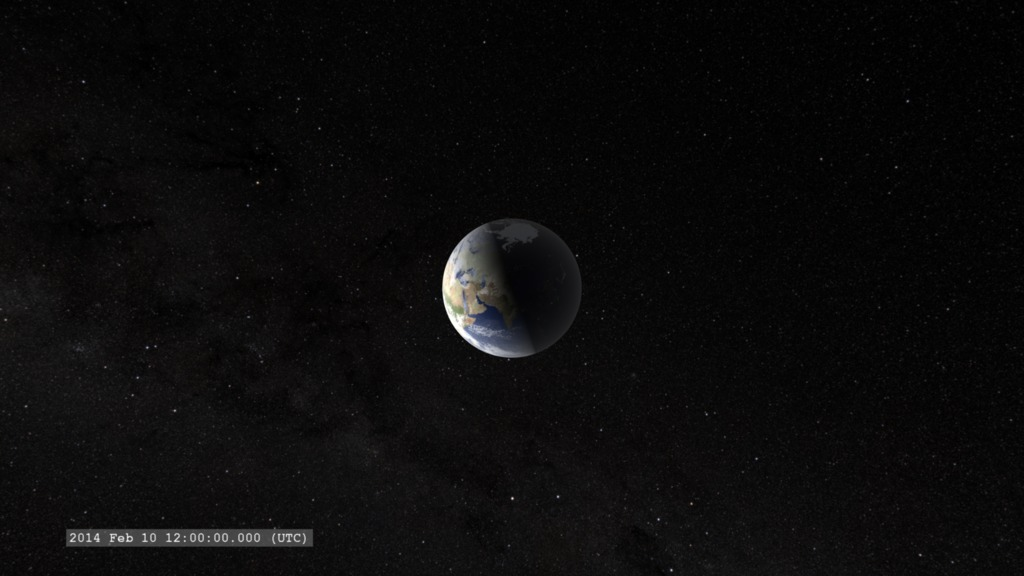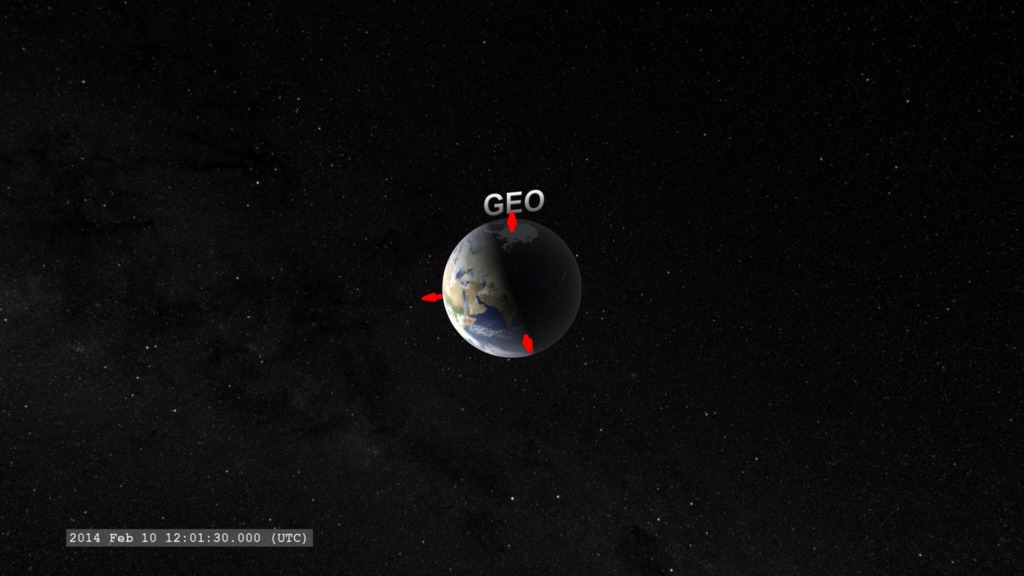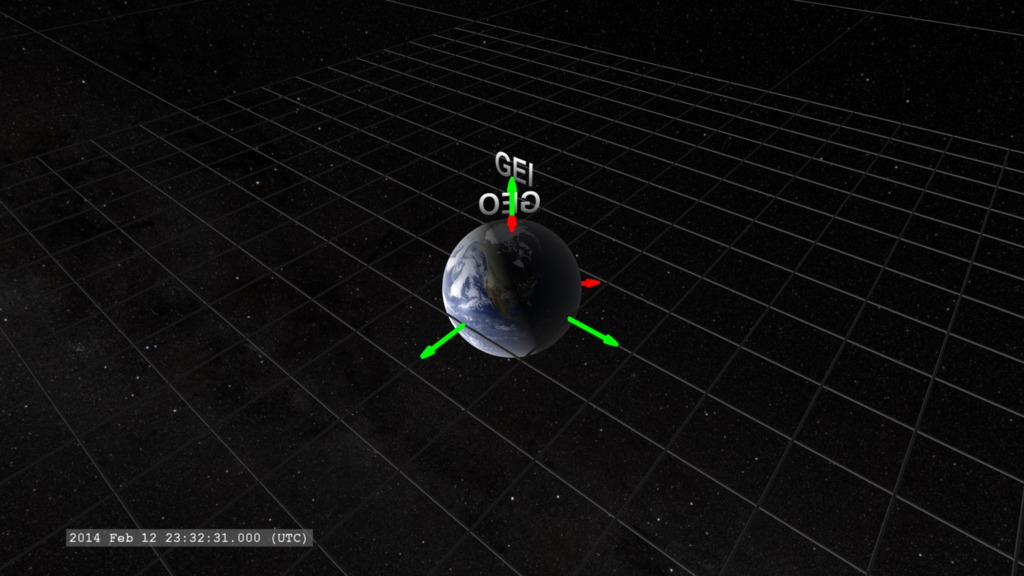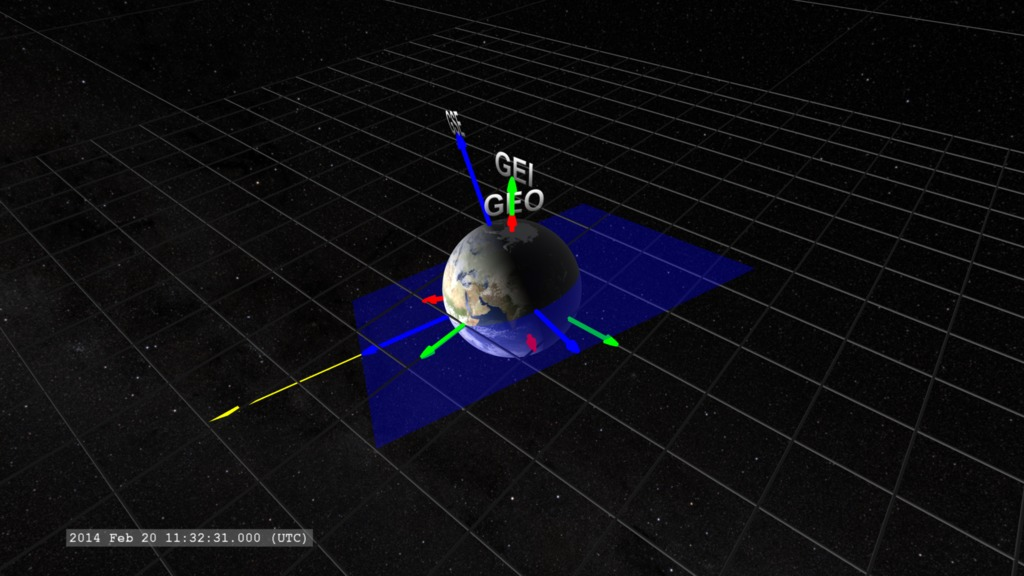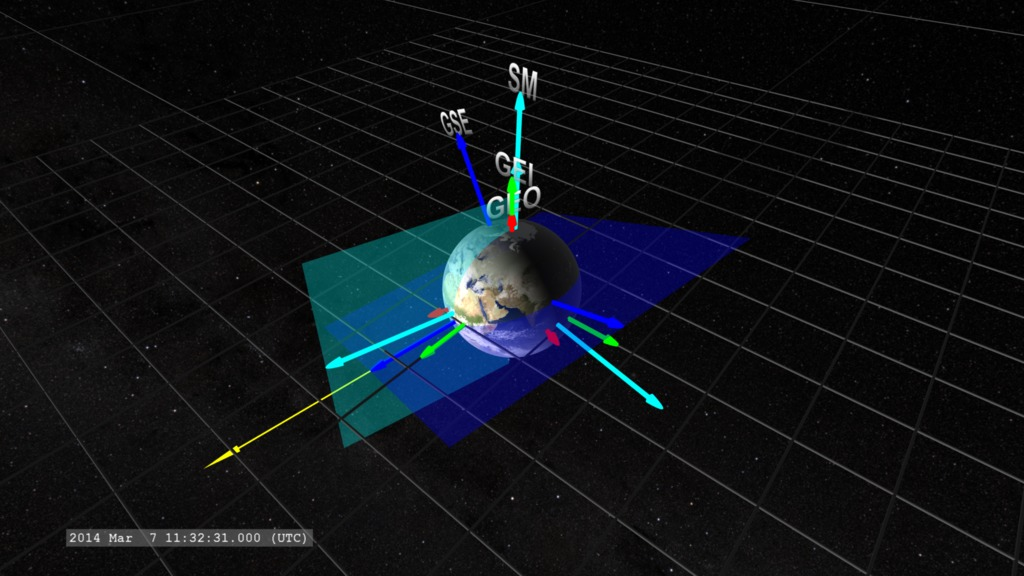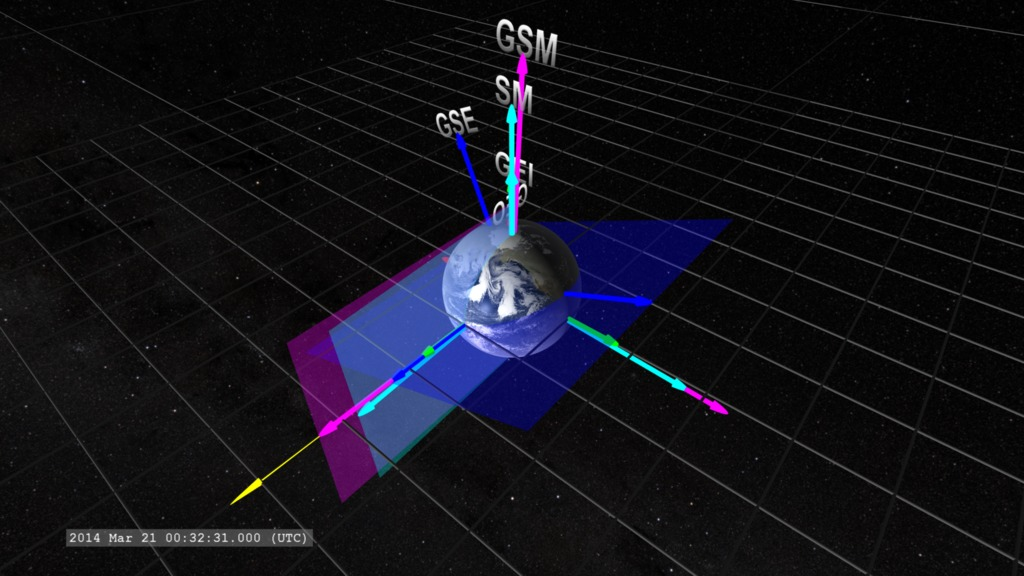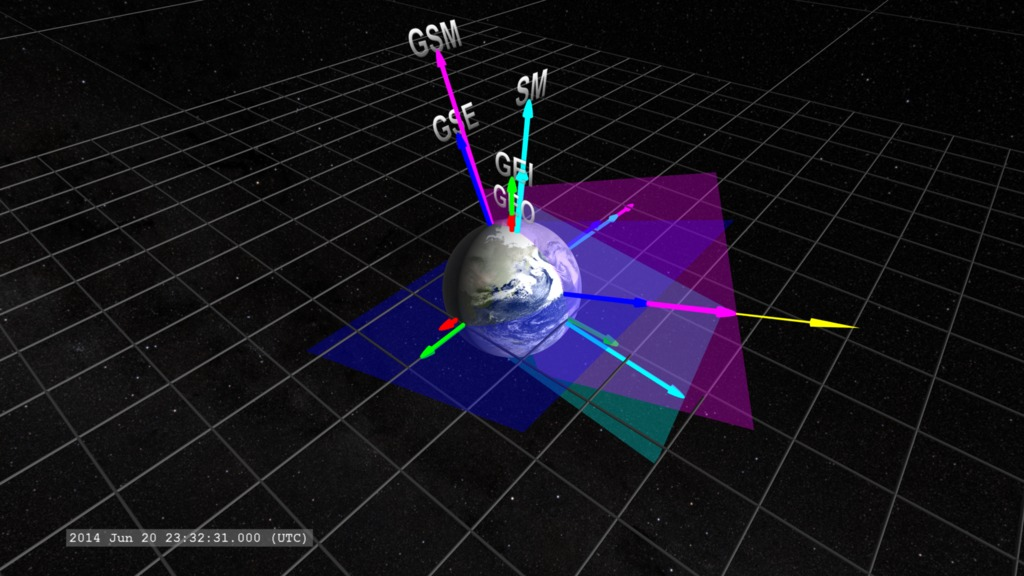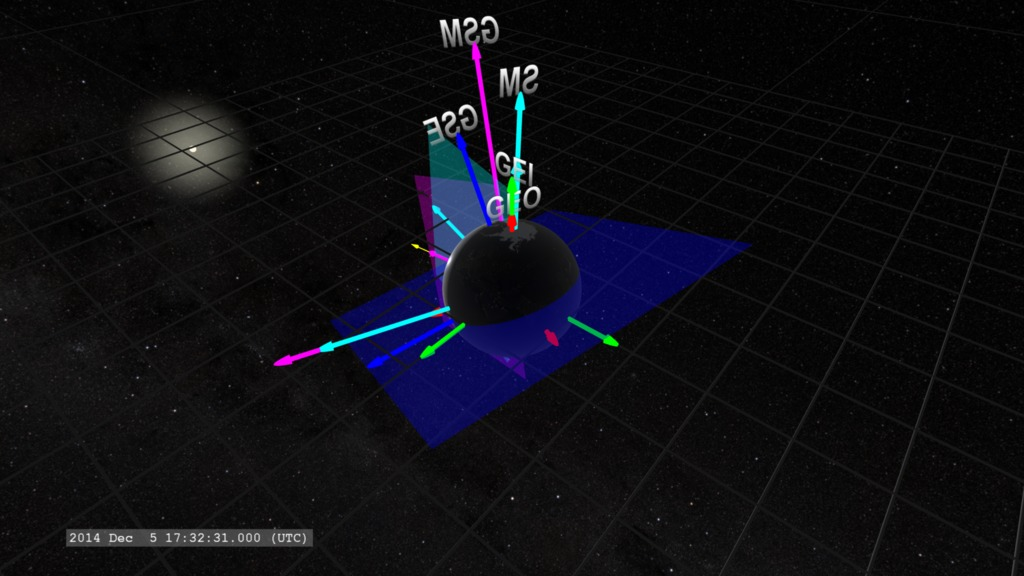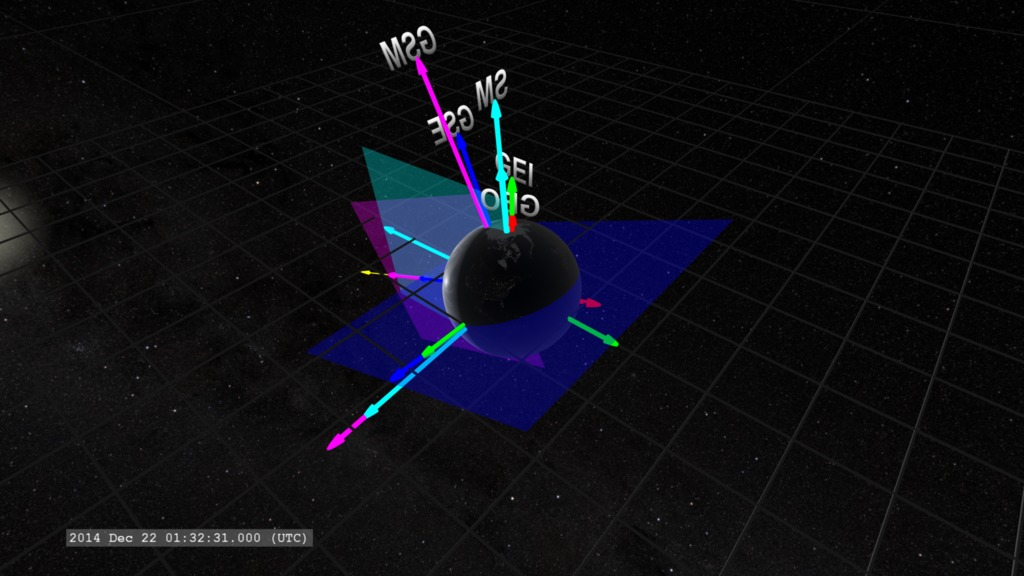Sun  ID: 4217

# Coordinated Earth: Measuring Space in the Near-Earth Environment

When we operate satellites in space, they are often taking measurements along the locations of their travel. As with many measurements, they are only useful if they can be placed in the proper context - their relationship to other measurements at the same, and different, locations. To assemble these measurements within context, we also need to know where and when the measurements were taken, and to do that, we need to define a coordinate system.

In three-dimensional space, we define a position with three numbers, relative to a point we define as the Origin of the coordinate system, defined as (0,0,0). Each number represents a distance from the origin along one of three directions. We usually defined these directions by axes, labelled X, Y, and Z, which are defined to be mutually perpendicular, each one is at right angles to the others.

While all coordinate systems are equal, all coordinate systems are not equally convenient for a given problem of interest. Sometimes the data and mathematics we use for exploring different problems can be more complex in one coordinate system or another. To simplify this, we often define a number of different coordinate systems and ways to do transformations between them.

In studying the space environment around Earth, we find five different coordinate systems of use.

• Geocentric (GEO): This is the coordinate system useful for measuring things close to Earth’s surface. The origin is chosen at the center of Earth. The x-axis points from the center of Earth through the Prime Meridian (by convention chosen as the meridian in Greenwich, London, UK (longitude = 0). The z-axis points towards the north geographic pole.

• Geocentric Earth Inertial (GEI): This coordinate system is fixed relative to the distant stars, so Earth rotates about the z-axis relative to it. The origin of this coordinate system is at the center of the Earth. The x-axis points to the first point in Aries (Wikipedia: Vernal Equinox) and the z-axis points to the north geographic & celestial pole. The direction of the celestial pole changes due to Earth’s rotational precession (Wikipedia).

• Geocentric Solar Ecliptic (GSE): The origin is at the center of the Earth. The x-axis is along the line between Earth and the Sun. The z-axis is the north ecliptic pole and is fixed in direction (but for slow changes due to Earth orbital changes).

• Solar Magnetic (SM): the origin is at the center of the Earth. The z-axis is chosen parallel to the Earth magnetic dipole axis. The y-axis is chosen to be perpendicular to the z-axis and the Earth-Sun line (pointing towards dusk).

• Geocentric Solar Magnetospheric (GSM): The origin is at the center of the Earth. The x-axis is defined as the Earth-Sun line (same as in GSE). The y-axis is defined to be perpendicular to the plane containing the x-axis and the magnetic dipole axis so the magnetic axis always lies in this plane.

Similar coordinate systems are defined for the Sun and other planets of the Solar System.

Development Note: This visualization was originally developed to test coordinate system transformations in the visualization framework.
References: